# Selina Solutions Concise Maths Class 10 Chapter 15 Similarity (With Applications to Maps and Models) Exercise 15(B)

Basic Proportionality theorem and its converse are widely discussed in this exercise. The problems of this exercise are well framed in order to give a deep understanding of this theorem. Selina Solutions for Class 10 Maths can be utilised by students to clarify any doubts in solving problems. All the solutions are developed by subject matter experts having vast academic experience. Avail Selina Solutions Concise Maths Class 10 Chapter 15 Similarity (With Applications to Maps and Models) Exercise 15(B) PDF, from the links provided below.

## Selina Solutions Concise Maths Class 10 Chapter 15 Similarity (With Applications to Maps and Models) Exercise 15(B) Download PDF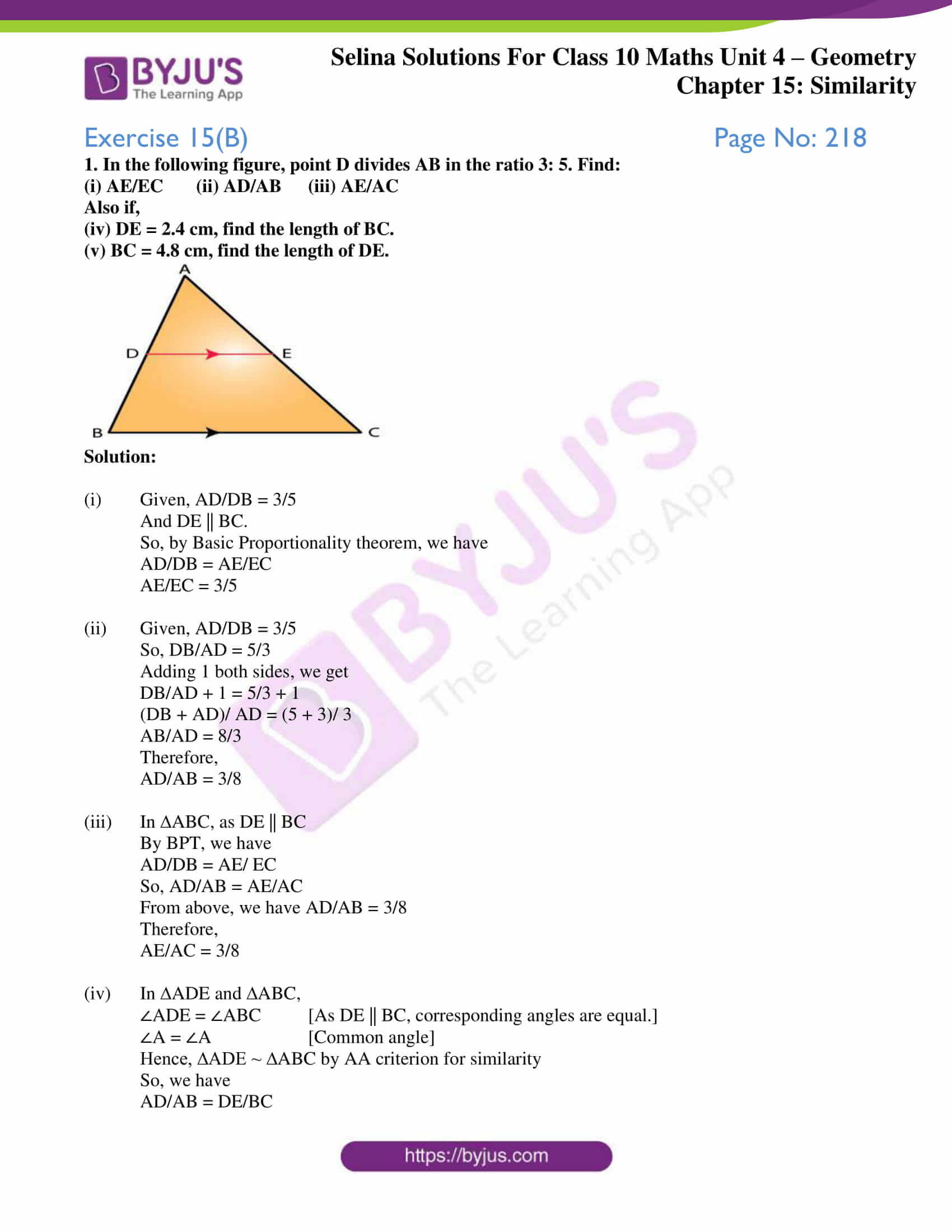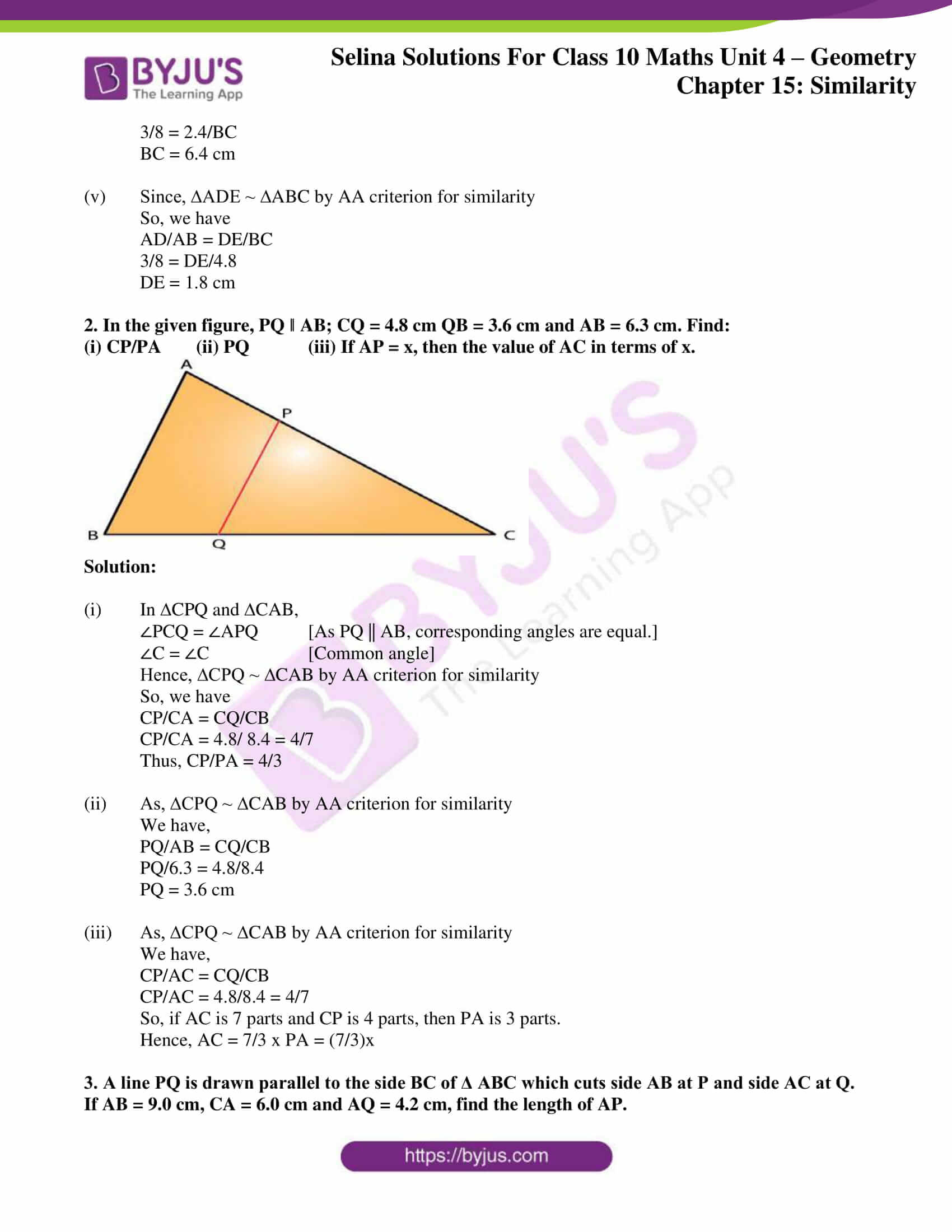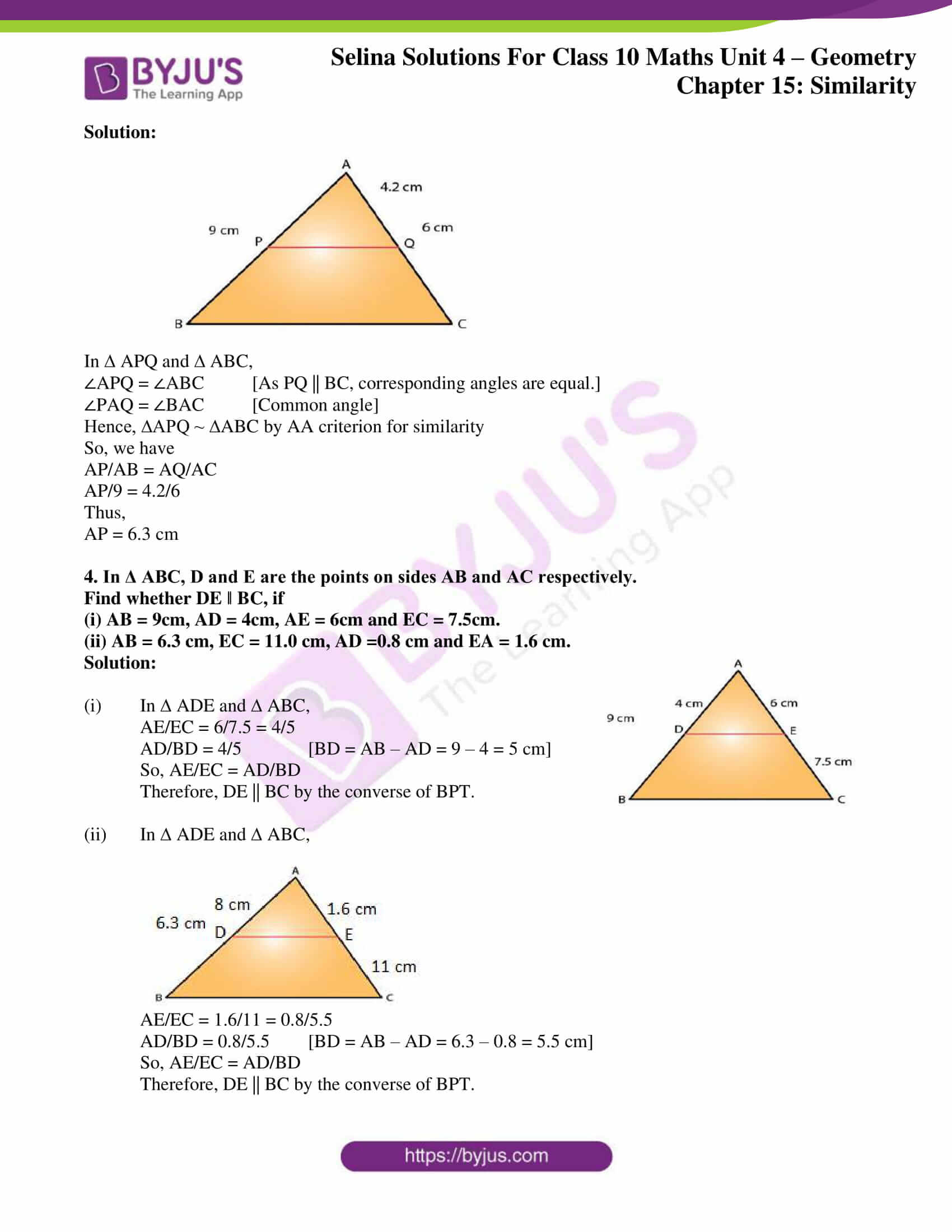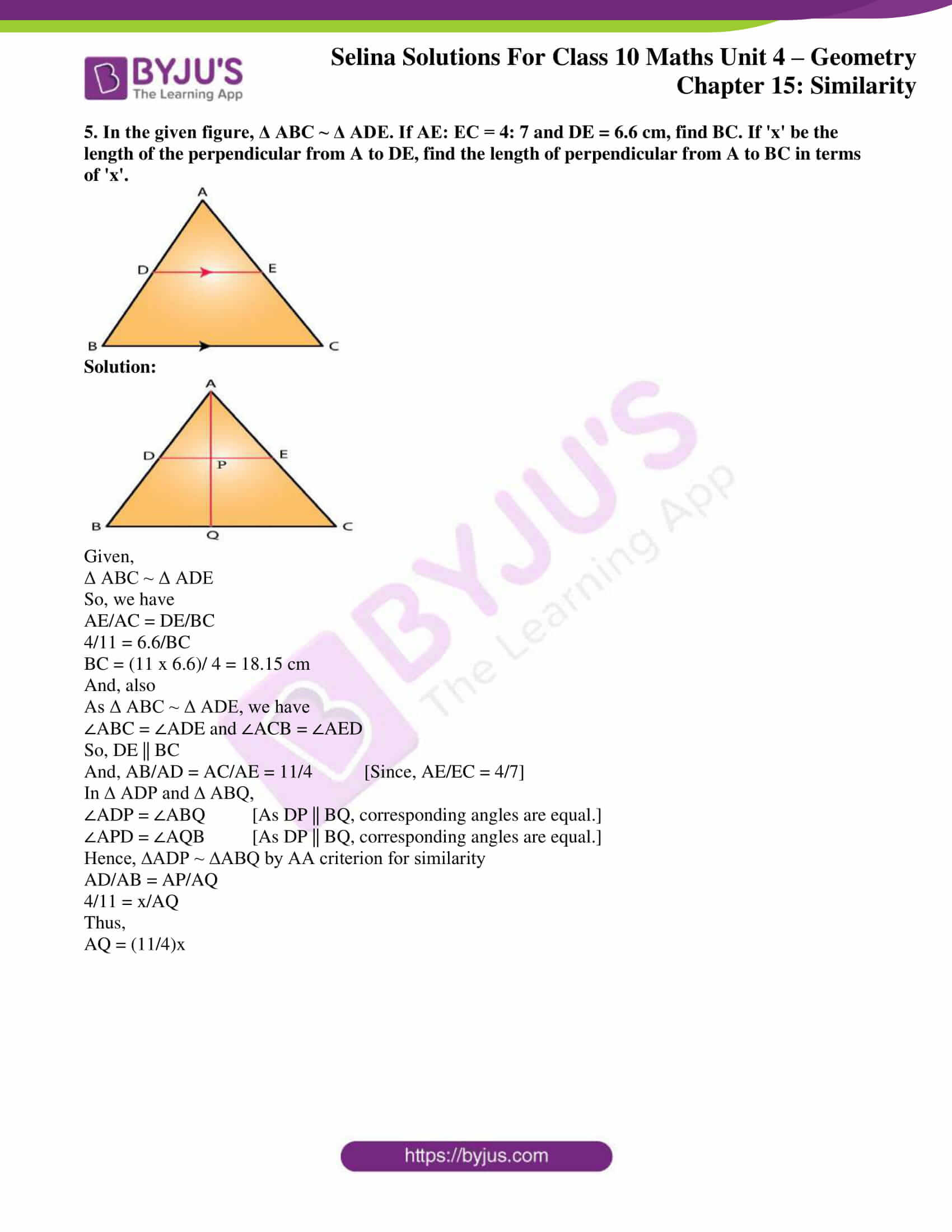## Access Selina Solutions Concise Maths Class 10 Chapter 15 Similarity (With Applications to Maps and Models)

#### Exercise 15(B) Page No: 218

1. In the following figure, point D divides AB in the ratio 3: 5. Find:

(i) AE/EC (ii) AD/AB (iii) AE/AC

Also if,

(iv) DE = 2.4 cm, find the length of BC.

(v) BC = 4.8 cm, find the length of DE.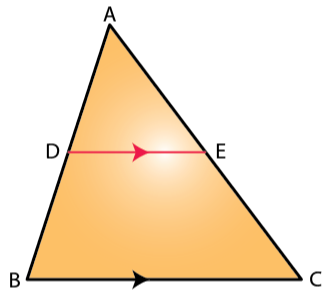Solution:

And DE || BC.

So, by Basic Proportionality theorem, we have

AE/EC = 3/5

Adding 1 both sides, we get

DB/AD + 1 = 5/3 + 1

Therefore,

(iii) In ∆ABC, as DE || BC

By BPT, we have

From above, we have AD/AB = 3/8

Therefore,

AE/AC = 3/8

∠ADE = ∠ABC [As DE || BC, corresponding angles are equal.]

∠A = ∠A [Common angle]

Hence, ∆ADE ~ ∆ABC by AA criterion for similarity

So, we have

3/8 = 2.4/BC

BC = 6.4 cm

(v) Since, ∆ADE ~ ∆ABC by AA criterion for similarity

So, we have

3/8 = DE/4.8

DE = 1.8 cm

2. In the given figure, PQ ‖ AB; CQ = 4.8 cm QB = 3.6 cm and AB = 6.3 cm. Find:

(i) CP/PA (ii) PQ (iii) If AP = x, then the value of AC in terms of x.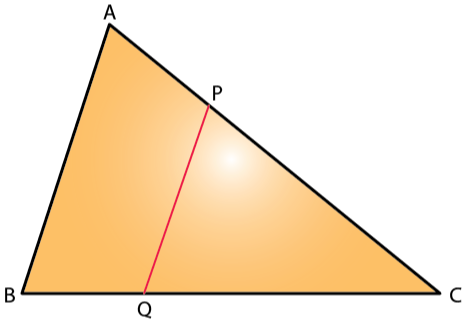Solution:

(i) In ∆CPQ and ∆CAB,

∠PCQ = ∠APQ [As PQ || AB, corresponding angles are equal.]

∠C = ∠C [Common angle]

Hence, ∆CPQ ~ ∆CAB by AA criterion for similarity

So, we have

CP/CA = CQ/CB

CP/CA = 4.8/ 8.4 = 4/7

Thus, CP/PA = 4/3

(ii) As, ∆CPQ ~ ∆CAB by AA criterion for similarity

We have,

PQ/AB = CQ/CB

PQ/6.3 = 4.8/8.4

PQ = 3.6 cm

(iii) As, ∆CPQ ~ ∆CAB by AA criterion for similarity

We have,

CP/AC = CQ/CB

CP/AC = 4.8/8.4 = 4/7

So, if AC is 7 parts and CP is 4 parts, then PA is 3 parts.

Hence, AC = 7/3 x PA = (7/3)x

3. A line PQ is drawn parallel to the side BC of Δ ABC which cuts side AB at P and side AC at Q. If AB = 9.0 cm, CA = 6.0 cm and AQ = 4.2 cm, find the length of AP.

Solution: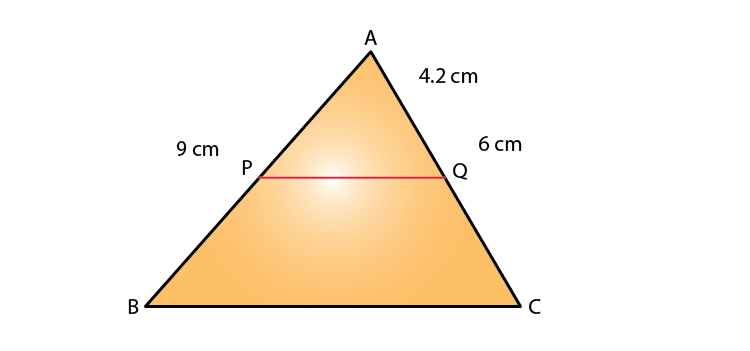In ∆ APQ and ∆ ABC,

∠APQ = ∠ABC [As PQ || BC, corresponding angles are equal.]

∠PAQ = ∠BAC [Common angle]

Hence, ∆APQ ~ ∆ABC by AA criterion for similarity

So, we have

AP/AB = AQ/AC

AP/9 = 4.2/6

Thus,

AP = 6.3 cm

4. In Δ ABC, D and E are the points on sides AB and AC respectively.

Find whether DE ‖ BC, if

(i) AB = 9cm, AD = 4cm, AE = 6cm and EC = 7.5cm.

(ii) AB = 6.3 cm, EC = 11.0 cm, AD =0.8 cm and EA = 1.6 cm.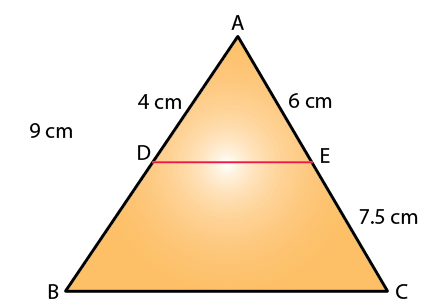Solution:

(i) In ∆ ADE and ∆ ABC,

AE/EC = 6/7.5 = 4/5

AD/BD = 4/5 [BD = AB – AD = 9 – 4 = 5 cm]

Therefore, DE || BC by the converse of BPT.

(ii) In ∆ ADE and ∆ ABC,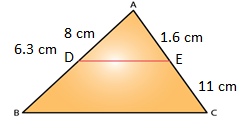AE/EC = 1.6/11 = 0.8/5.5

AD/BD = 0.8/5.5 [BD = AB – AD = 6.3 – 0.8 = 5.5 cm]

Therefore, DE || BC by the converse of BPT.

5. In the given figure, Δ ABC ~ Δ ADE. If AE: EC = 4: 7 and DE = 6.6 cm, find BC. If ‘x’ be the length of the perpendicular from A to DE, find the length of perpendicular from A to BC in terms of ‘x’.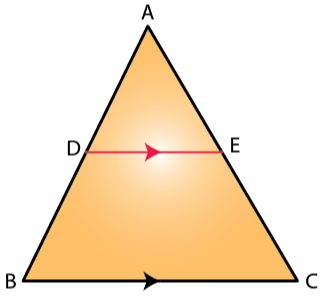Solution: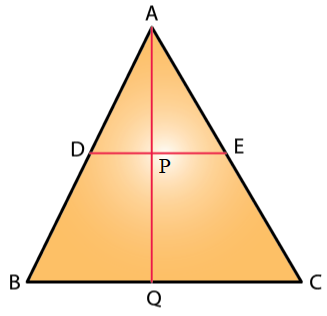Given,

So, we have

AE/AC = DE/BC

4/11 = 6.6/BC

BC = (11 x 6.6)/ 4 = 18.15 cm

And, also

As Δ ABC ~ Δ ADE, we have

∠ABC = ∠ADE and ∠ACB = ∠AED

So, DE || BC

And, AB/AD = AC/AE = 11/4 [Since, AE/EC = 4/7]

In ∆ ADP and ∆ ABQ,

∠ADP = ∠ABQ [As DP || BQ, corresponding angles are equal.]

∠APD = ∠AQB [As DP || BQ, corresponding angles are equal.]

Hence, ∆ADP ~ ∆ABQ by AA criterion for similarity

4/11 = x/AQ

Thus,

AQ = (11/4)x

### Access other exercises of Selina Solutions Concise Maths Class 10 Chapter 15 Similarity (With Applications to Maps and Models)

Exercise 15(A) Solutions

Exercise 15(C) Solutions

Exercise 15(D) Solutions

Exercise 15(E) Solutions